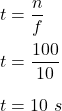## A ball is spun around in circular motion such that its frequency is 10 Hz. a. What is the period of its rotation? b. How much ti

Question

A ball is spun around in circular motion such that its frequency is 10 Hz.
a. What is the period of its rotation?
b. How much time will be required to complete 100 rotations?

in progress 0
2 months 2021-07-21T10:18:29+00:00 1 Answers 3 views 0

a = 0.1 s b. 10 s

Explanation:

Given that,

The frequency in circular motion, f = 10 Hz

(a) Let T is the period of itsrotation. We know that,

T = 1/f

So,

T = 1/10

= 0.1 s

(b) Frequency is number of rotations per unit time. So,Hence, this is the required solution.